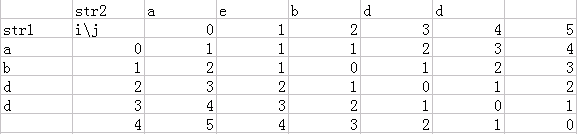# 计算字符串的相似度--编程之美3.3

1.修改一个字符（如把“a”替换为“b”）;

2.增加一个字符（如把“abdd”变为“aebdd”）;

3.删除一个字符（如把“travelling”变为“traveling”）;

比如，对于“abcdefg”和“abcdef”两个字符串来说，我们认为可以通过增加/减少一个“g”的方式来达到目的。上面的两种方案，都仅需要一 次 。把这个操作所需要的次数定义为两个字符串的距离，而相似度等于“距离+1”的倒数。也就是说，“abcdefg”和“abcdef”的距离为1，相似度 为1/2=0.5。

给定任意两个字符串，你是否能写出一个算法来计算它们的相似度呢？

原文的分析与解法

不难看出，两个字符串的距离肯定不超过它们的长度之和（我们可以通过删除操作把两个串都转化为空串）。虽然这个结论对结果没有帮助，但至少可以知道，任意两个字符串的距离都是有限的。

我们还是就住集中考虑如何才能把这个问题转化成规模较小的同样的子问题。如果有两个串A=xabcdae和B=xfdfa，它们的第一个字符是 相同的，只要计算A[2,...,7]=abcdae和B[2,...,5]=fdfa的距离就可以了。但是如果两个串的第一个字符不相同，那么可以进行 如下的操作（lenA和lenB分别是A串和B串的长度）。

1.删除A串的第一个字符，然后计算A[2,...,lenA]和B[1,...,lenB]的距离。

2.删除B串的第一个字符，然后计算A[1,...,lenA]和B[2,...,lenB]的距离。

3.修改A串的第一个字符为B串的第一个字符，然后计算A[2,...,lenA]和B[2,...,lenB]的距离。

4.修改B串的第一个字符为A串的第一个字符，然后计算A[2,...,lenA]和B[2,...,lenB]的距离。

5.增加B串的第一个字符到A串的第一个字符之前，然后计算A[1,...,lenA]和B[2,...,lenB]的距离。

6.增加A串的第一个字符到B串的第一个字符之前，然后计算A[2,...,lenA]和B[1,...,lenB]的距离。

在这个题目中，我们并不在乎两个字符串变得相等之后的字符串是怎样的。所以，可以将上面的6个操作合并为：

1.一步操作之后，再将A[2,...,lenA]和B[1,...,lenB]变成相字符串。

2.一步操作之后，再将A[2,...,lenA]和B[2,...,lenB]变成相字符串。

3.一步操作之后，再将A[1,...,lenA]和B[2,...,lenB]变成相字符串。

这样，很快就可以完成一个递归程序。

原文算法代码View Code
 int calculateStringDistance(string strA, int pABegin, int pAEnd, string strB, int pBBegin, int pBEnd) {　　　　 if(pABegin > pAEnd)　　　　 {　　　　　　　　 if(pBBegin > pBEnd)　　　　　　　　　　　　 return 0;　　　　　　　　 else　　　　　　　　　　　　 return pBEnd - pBBegin + 1;　　　　 }　　　　 if(pBBegin > pBEnd)　　　　 {　　　　　　　　 if(pABegin > pAEnd)　　　　　　　　　　　　 return 0;　　　　　　　　 else　　　　　　　　　　　　 return pAEnd - pABegin + 1;　　　　 }　　　　 if(strA[pABegin] == strB[pBBegin])　　　　 {　　　　　　　　 return calculateStringDistance(strA, pABegin+1, pAEnd, strB, pBBegin+1, pBEnd);　　　　 }　　　　 else　　　　 {　　　　　　　　 int t1 = calculateStringDistance(strA, pABegin, pAEnd, strB, pBBegin+1, pBEnd);　　　　　　　　 int t2 = calculateStringDistance(strA, pABegin+1, pAEnd, strB, pBBegin, pBEnd);　　　　　　　　 int t3 = calculateStringDistance(strA, pABegin+1, pAEnd, strB, pBBegin+1, pBEnd);　　　　　　　　 return minValue(t1, t2, t3) + 1;　　　　 } }

我们知道适合采用动态规划方法的最优化问题中的两个要素：最优子结构和重叠子问题。另外，还有一种方法称为备忘录（memoization），可以充分利用重叠子问题的性质。

下面简述一下动态规划的基本思想。和分治法一样，动态规划是通过组合子问题的解而解决整个问题的。我们知道，分治算法是指将问题划分 成一睦独立的子问题，递归 地求解各子问题，然后合并子问题的解而得到原问题的解。与此不同，动态规划适用于子问题不是独立 的情况，也就是各子问题包含公共的子子问题。在这种情况 下，若用分治法则会做许多不必要的工作，即重复地求解公共的子子问题。动态规划算法对每个子子问题只求解一次，将其结果保存在一张表中，从而避免每次遇到 各个子问题时重新计算答案。

动态规划算法的设计可以分为如下4个步骤：

1）描述最优解的结构。

2）递归定义最优解的值。

3）按自底向上的方式计算最优解的值。

4）由计算出的结果构造一个最优解。

第1~3步构成问题的动态规划解的基础。第4步在只要求计算最优解的值时可以略去。如果的确做了第4步，则有时要在第3步的计算中记录一些附加信息，使构造一个最优解变得容易。

该问题明显完全符合动态规划的两个要素，即最优子结构和重叠子问题特性。该问题的最优指的是两个字符串的最短距离，子问题的重叠性可以从原书中的那个递归算法中看出。

下面再来详细说说什么是重叠子问题。适用于动态规划求解的最优化问题必须具有的第二个要素是子问题的空间要“很小”，也就是用来解原问题的递归 算法可以反复地解同样的子问题，而不是总在产生新的子问题。典型地，不同的子问题数是输入规模的一个多项式。当一个递归算法不断地调用同一问题时，我们说 该最优问题包含重叠子问题。相反地，适合用分治法解决的问题只往往在递归的每一步都产生全新的问题。动态规划算法总是充分利用重叠子问题，即通过每个子问 题只解一次，把解保存在一个需要时就可以查看的表中，而每次查表的时间为常数。View Code
/*DP Algorithm　 * A loop method using dynamic programming.　 * Calculate from bottom to top.　 */ int calculateStringDistance(string strA, string strB) {　　　　 int lenA = (int)strA.length();　　　　 int lenB = (int)strB.length();　　　　 int c[lenA+1][lenB+1];         // Record the distance of all begin points of each string        //初始化方式与背包问题有点不同　　　　 for(int i = 0; i < lenA; i++) c[i][lenB] = lenA - i;　　　　 for(int j = 0; j < lenB; j++) c[lenA][j] = lenB - j;　　　　 c[lenA][lenB] = 0;　　　　 for(int i = lenA-1; i >= 0; i--)　　　　　　　　 for(int j = lenB-1; j >= 0; j--)　　　　　　　　 {　　　　　　　　　　　　 if(strB[j] == strA[i])　　　　　　　　　　　　　　　　 c[i][j] = c[i+1][j+1];　　　　　　　　　　　　 else　　　　　　　　　　　　　　　　 c[i][j] = minValue(c[i][j+1], c[i+1][j], c[i+1][j+1]) + 1;　　　　　　　　 } 　　　　 return c; }加了备忘的递归算法为每一个子问题的解在表中记录一个表项。开始时，每个表项最初都包含一个特殊的值，以表示该表项有待填入。当在递归算法的执行中第一次遇到一个子问题时，就计算它的解并填入表中。以后每次遇到该子问题时，只要查看并返回先前填入的值即可。

下面是原文递归算法的做备忘录版本，并通过布尔变量memoize来控制是否使用备忘录，以及布尔变量debug来控制是否打印调用过程。有兴趣的读都可以通过这两个布尔变量的控制来对比一下备忘录版本与非备忘录版本的复杂度。

备忘录版View Code
 #include <iostream> #define M 100  using namespace std;  const bool debug = false; // Whether to print debug info const bool memoize = true; // Whether to use memoization unsigned int cnt = 0; // Line number for the debug info  int memoizedDistance[M][M]; // Matrix for memoiztion  int minValue(int a, int b, int c) {　　　　 if(a < b && a < c) return a;　　　　 else if(b < a && b < c) return b;　　　　 else return c; }  /*　20　 * A recursive method which can be decorated by memoization.　 * Calculate from top to bottom. */ int calculateStringDistance(string strA, int pABegin, int pAEnd, string strB, int pBBegin, int pBEnd) {　　　　 if(memoize && memoizedDistance[pABegin][pBBegin] >= 0)　　　　　　　　 return memoizedDistance[pABegin][pBBegin]; 　　　　 if(pABegin > pAEnd)　　　　 {　　　　　　　　 if(pBBegin > pBEnd)　　　　　　　　 {　　　　　　　　　　　　 if(memoize)　　　　　　　　　　　　　　　　 memoizedDistance[pABegin][pBBegin] = 0;　　　　　　　　　　　　 if(debug)　　　　　　　　　　　　　　　　 cout << cnt++ << ": m(" << pABegin << "," << pBBegin << ")=0" << endl;　　　　　　　　　　　　 return 0;　　　　　　　　 }　　　　　　　　 else　　　　　　　　 {　　　　　　　　　　　　 int temp = pBEnd - pBBegin + 1;　　　　　　　　　　　　 if(memoize)　　　　　　　　　　　　　　　　 memoizedDistance[pABegin][pBBegin] = temp;　　　　　　　　　　　　 if(debug)　　　　　　　　　　　　　　　　 cout << cnt++ << ": m(" << pABegin << "," << pBBegin << ")=" << temp << endl;　　　　　　　　　　　　 return temp;　　　　　　　　 }　　　　 } 　　　　 if(pBBegin > pBEnd)　　　　 {　　　　　　　　 if(pABegin > pAEnd)　　　　　　　　 {　　　　　　　　　　　　 if(memoize)　　　　　　　　　　　　　　　　 memoizedDistance[pABegin][pBBegin] = 0;　　　　　　　　　　　　 if(debug)　　　　　　　　　　　　　　　　 cout << cnt++ << ": m(" << pABegin << "," << pBBegin << ")=0" << endl;　　　　　　　　　　　　 return 0;　　　　　　　　 }　　　　　　　　 else　　　　　　　　 {　　　　　　　　　　　　 int temp = pAEnd - pABegin + 1;　　　　　　　　　　　　 if(memoize)　　　　　　　　　　　　　　　　 memoizedDistance[pABegin][pBBegin] = temp;　　　　　　　　　　　　 if(debug)　　　　　　　　　　　　　　　　 cout << cnt++ << ": m(" << pABegin << "," << pBBegin << ")=" << temp << endl;　　　　　　　　　　　　 return temp;　　　　　　　　 }　　　　 } 　　　　 if(strA[pABegin] == strB[pBBegin])　　　　 {　　　　　　　　 int temp = calculateStringDistance(strA, pABegin+1, pAEnd, strB, pBBegin+1, pBEnd);　　　　　　　　 if(memoize)　　　　　　　　　　　　 memoizedDistance[pABegin][pBBegin] = temp;　　　　　　　　　 if(debug)　　　　　　　　　　　　 cout << cnt++ << ": m(" << pABegin << "," << pBBegin << ")=" << temp << endl;　　　　　　　　 return temp;　　　　 }　　　　 else　　　　 {　　　　　　　　 int t1 = calculateStringDistance(strA, pABegin, pAEnd, strB, pBBegin+1, pBEnd);　　　　　　　　 int t2 = calculateStringDistance(strA, pABegin+1, pAEnd, strB, pBBegin, pBEnd);　　　　　　　　 int t3 = calculateStringDistance(strA, pABegin+1, pAEnd, strB, pBBegin+1, pBEnd);　　　　　　　　 int temp = minValue(t1, t2, t3) + 1;　　　　　　　　 if(memoize)　　　　　　　　　　　　 memoizedDistance[pABegin][pBBegin] = temp;　　　　　　 if(debug)　　　　　　　　　　　　 cout << cnt++ << ": m(" << pABegin << "," << pBBegin << ")=" << temp << endl;　　　　　　　　 return temp;　　　　 } }  int main() {　　　　 if(memoize)　　　　 {　　　　　　　　 // initialize the matrix : memoizedDistance[][]　　　　　　　　 for(int i = 0; i < M; i++)　　　　　　　　　　　　 for(int j = 0; j < M; j++)　　　　　　　　　　　　　　　　 memoizedDistance[i][j] = -1; // -1 means unfilled cell yet　　　　 } 　　　　 string strA = "abcdfef";　　　　 string strB = "a"; 　　　　 cout << endl << "Similarity = "　　　　　　　　　　　　 << 1.0 / (1 + calculateStringDistance(strA, 0, (int)strA.length()-1, strB, 0, (int)strB.length()-1))　　　　　　　　　　　　 << endl; 　　　　 return 0; }

在实际应用中，如果所有的子问题都至少要被计算一次，则一个自底向上的动态规划算法通常要比一个自顶向下的做备忘录算法好出一个常数因子，因为前者无需递归的代价，而且维护表格的开销也小些。此外，在有些问题中，还可以用动态规划算法中的表存取模式来进一步减少时间或空间上的需求。或者，如果子问题空间中的某些子问题根本没有必要求解，做备忘录方法有着只解那些肯定要求解的子问题的优点，对于本问题就是这样。

posted on 2011-04-03 20:16 tzc_yujunyong 阅读(...) 评论(...)  编辑 收藏